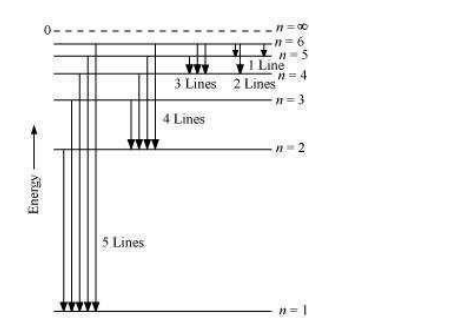# What is the maximum number of emission lines when the excited electron of an HQuestion.

What is the maximum number of emission lines when the excited electron of an H atom in n = 6 drops to the ground state?

Solution:

When the excited electron of an H atom in n = 6 drops to the ground state, the following transitions are possible:Hence, a total number of (5 + 4 + 3 + 2 + 1) 15 lines will be obtained in the emission spectrum.

The number of spectral lines produced when an electron in the $n^{\text {th }}$ level drops down to

the ground state is given by $\frac{n(n-1)}{2}$

Given, n = 6

Number of spectral lines $=\frac{6(6-1)}{2}=15$